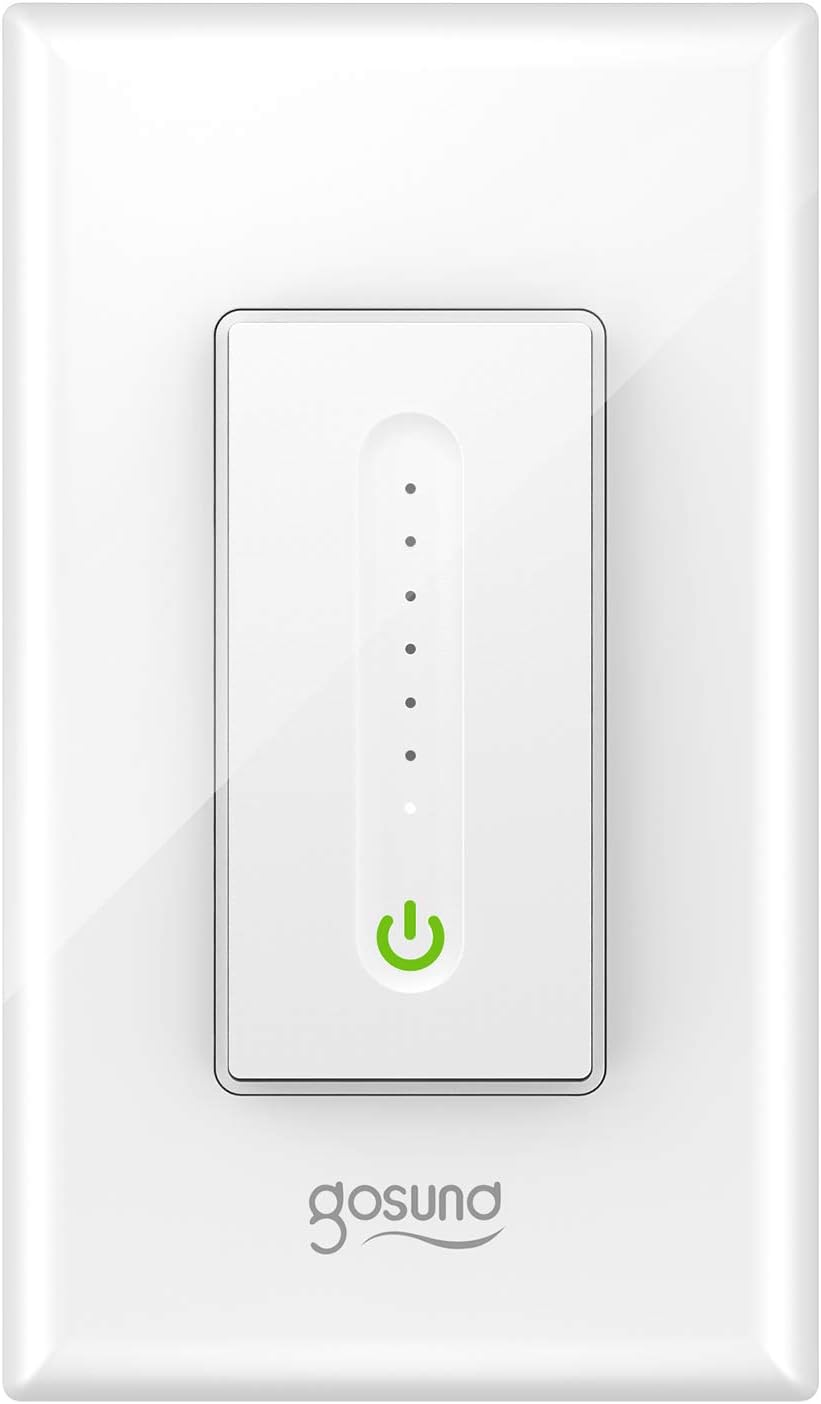## Gosund SW2 DimmerAvailable from:

Manufacturer:

Flashed with:

GPIO #Component
GPIO00 User
GPIO01 Serial Tx
GPIO02 User
GPIO03 Serial Rx
GPIO04 Button1
GPIO05 None
GPIO09 User
GPIO10 User
GPIO12 Led1i
GPIO14 PWM1
GPIO15 User
GPIO16 User
FLAG None

#### Template

``{"NAME":"Gosund Dimmer","GPIO":[255,148,255,149,17,0,255,255,56,158,37,255,255],"FLAG":0,"BASE":18}``

This setup requires enabling Scripting

All dimming and on/off functionality is controlled by an MCU that does not follow the Tuya protocol. Dimming and on/off can be set by Tasmota using simple serial commands. Reading local dimming when set by the capacitive touch panel requires a Script-enabled build and the script below to process serial status codes sent by the dimmer MCU.

Status codes take the form of 24xx012123, with xx ranging from 01 to 64 (hex 1-100).

Minimum dimming level may vary by light fixture - adjust the ‘off’ value to match your setup in the >D section.

``````>D 100
rng=235
dimh=""
slider=0
power=0
dimval=1
a=""
b=""
c=""
d=0
f=0
;set the hex code below to the largest value possible that will turn off the relay with SerialSend5
off=0x7f

>B
dp0
rng-=off
=#scDim(dimval)

>E
slider=Dimmer
if chg[slider]>0
then
; dim according slider
if slider>0 and dimval!=slider
then
dimval=slider
=#scDim(dimval)
=>SerialSend5 %dimh%
endif
endif

if pwr!=power
then
if pwr==1
then
=>SerialSend5 %dimh%
power=1
else
=>Serialsend5 00
power=0
endif
endif

if sl(a)>0
then
;process the first and second hex nibbles
b=sb(a -8 1)
c=sb(a -7 1)
a=""
;convert the first nibble to decimal – max value is 0x60
f=b
f*=16
;handle second nibble which may be 0x0-0xf
d=c
if d==0
then
switch c
case "A"
d=10
case "B"
d=11
case "C"
d=12
case "D"
d=13
case "E"
d=14
case "F"
d=15
ends
endif
;add first and second nibbles then to Tasmota dimming path
f+=d
=>dimmer %f%
endif

#scDim(dimval)
dimh=hn((rng*dimval/100)+off)
=>print Scaled brightness is %dimh%
``````